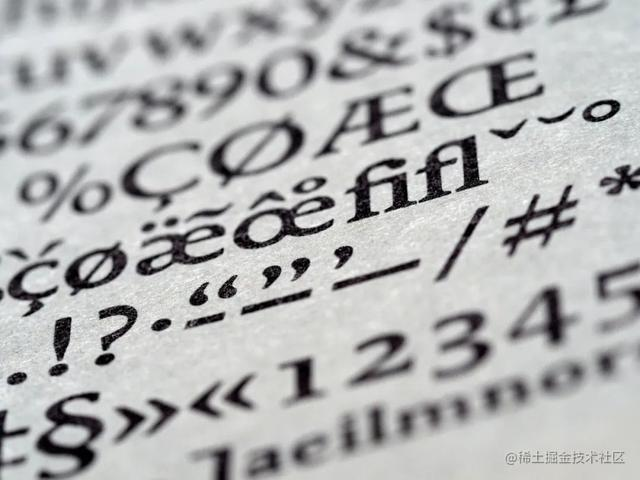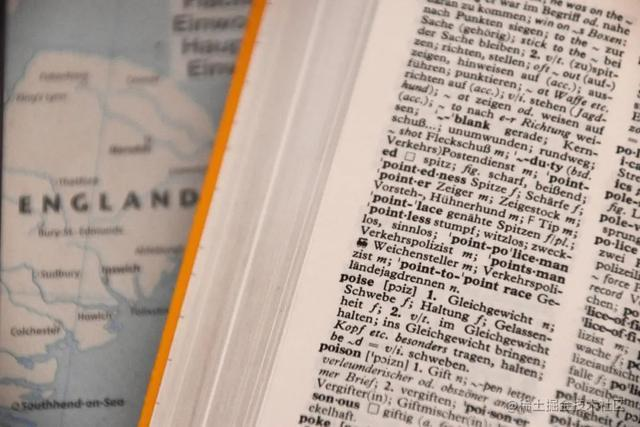# Python初学者必学的20个重要技巧Python是世界上使用最广泛的编程语言之一，原因有很多：易理解、用途非常广泛、包含了大量的模块和库等等。其中，简洁和较高的可读性使Python在所有软件中非常突出。

1.交换价值

"""valueswapping"""

a, b=5, 10

print(a, b)

a, b= b, a

print(a, b)

output

10, 5

2.列表中所有项的一个字符串

p = ["Python", "is", "a","popular", "language"]

print(" ".join(p))output

Python is a popular language

1. 列表中最常见的元素

list1 = [0, 1, 2, 3, 3, 2, 3, 1, 4, 5, 4]

print(max(set(list1), key = list1.count))output

3

4.测试两个字符串是否为相同字母异序词

defanagram(string_1,string_2):

"""Test if the stringsare anagrams.

string_1: string

string_2: string

returns: boolean

"""

from collections importCounter

defanagram(string_1,string_2):

returnCounter(string_1) ==Counter(string_2)

anagram('pqrs','rqsp')

True

anagram('pqrs','rqqs')

False5.逆转字符串

# with slicing

str ="PQRST"

reverse_str = str[::-1]

print(reverse_str)

Output

TSRQP

6.反转列表

# using slicing approach

defReverse(lst):

lst1 = lst[::-1]

return lst1

lst = [5, 6, 7, 8, 9, 10]

print(Reverse(lst))

output

[10, 9, 8, 7, 6, 5]

1. 转置矩阵

mat=[(5,6,7),(8,9,10),(11,12,13),(14,15,16)]

for row in mat:

print(row)

print("\n")

t_mat=zip(*mat)

for row in t_mat:

print(row)

output

(5, 6, 7)

(8, 9, 10)

(11, 12, 13)

(14, 15, 16)

(5, 8, 11, 14)

(6, 9, 12, 15)

(7, 10, 13, 16)

1. 链式比较

p < q< r

if p< q< r:

{.....}

# chaining comparison

a =3

print(1< a<10)

print(5< a<15)

print(a <7< a*7<49)

print(8> a<=6)

print(3== a>2)

output

True

False

True

True

True

1. ‘get’字典

dict = {"P":1, "Q":2}

print(dict["P"])

print(dict["R"])

Traceback (most recent call last):

File ".\dict.py", line 3, in

print (dict["R"])

KeyError: 'R'

dict = {"P":1, "Q":2}

print(dict.get("P"))

print(dict.get("R"))

print(dict.get("R","Unavailable! "))

output

1

None

Unavailable!

1. 按值给字典排序

defdict():

keyval ={}

# Initializing the value

keyval =48

keyval =6

keyval =10

keyval =22

keyval =15

keyval =245

"in alphabetical order by thevalue")

# Convert it to float to mathematicalpurposes

print(sorted(keyval.elements(), key =

lambda k_val:(k_val, k_val)))

defmain():

dict()

if name=="main":

main()

output

[(2, 6), (5, 10), (6, 15), (1, 22), (3, 48), (4, 245)]1. 列表推导

# Multiplying each item in the list with 3

list1 = [2,4,6,8]

list2 = [3*p for p in list1]

print(list2)

[6,12,18,24]

1. 执行部分计划所花费的时间

import time

initial_Time = time.time()

x, y=5,6

z = x+ y

# Program to test ending

ending_Time = time.time()

Time_lapsed_in_Micro_sec= (ending_Time- initial_Time)*(10**6)

print(" Timelapsed in micro_seconds: {0} ms").format(Time_lapsed_in_Micro_sec)

1. 合并字典

dic1 = {'men': 6, 'boy': 5}

dic2 = {'boy': 3, 'girl': 5}

merged_dic = {**dic1, **dic2}

print(merged_dic)

Output

{'men': 6, 'boy': 3, 'girl': 5}

1. 数字化

number =2468

# with map

digit_list =list(map(int, str(number)))

print(digit_list)

[2, 4, 6, 8]

# with list comprehension

digit_list = [int(a) for a instr(number)]

print(digit_list)

[2, 4, 6, 8]

# Even simpler approach

digit_list =list(str(number))

print(digit_list)

[2, 4, 6, 8]

1. 测试独特性

defuniq(list):

iflen(list)==len(set(list)):

print("totalitems are unique")

else:

print("Listincludes duplicate item")

uniq([0,2,4,6])

total items are unique

uniq([1,3,3,5])

List includesduplicate item

1. 使用枚举

sample_list = [4, 5, 6]

for j, item inenumerate(sample_list):

print(j, ': ', item)

Output

0 : 4

1 : 5

2 : 6

17.在一行中计算任意数的阶乘

import functools

fact = (lambda i: functools.reduce(int.mul, range(1,i+1),1)(4)

print(fact)

Output

24

1. 返回几个函数的元素

defa():

return5, 6, 7, 8

# Calling the abovefunction.

w, x, y, z=a()

print(w, x, y, z)

Output

5678

1. 加入一个真正的Python切换大小写语句

defaswitch(a):

returnaswitch._system_dic.get(a, None)

aswitch._system_dic = {'mangoes': 4, 'apples': 6, 'oranges': 8}

print(aswitch('default'))

print(aswitch('oranges'))

Output

None

8

20.使用splat操作符解包函数参数

splat操作符提供了一种解包参数列表的有效方法：

deftest(a, b, c):

print(p, q, r)

test_Dic = {'a': 4, 'b': 5, 'c': 6}

test_List = [10, 11, 12]

test(*test_Dic)

test(**test_Dic)

test(*test_List)

#1-> p q r

#2-> 4 5 6

#3-> 10 11 12